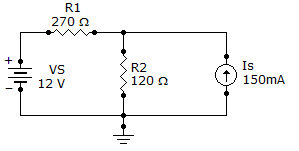# Online Electrical Engineering Test - Electrical Engineering Test 6Loading Test...

Instruction:

• This is a FREE online test. DO NOT pay money to anyone to attend this test.
• Total number of questions : 20.
• Time alloted : 30 minutes.
• Each question carry 1 mark, no negative marks.
• DO NOT refresh the page.
• All the best :-).

1.

A two-terminal variable resistor is known as a

A.
 potentiometerB.
 thermistorC.
 rheostatD.
 wiper2.

If 24 V are applied across a resistor and there are 10.9 mA of current, the resistance is

A.
 220 kB.
 22 kC.
 2.2 kD.
 2203.

How many watt-hours represent 65 W used for 18 h?

A.
 11.7 WhB.
 1,170 WhC.
 11,700 WhD.
 117,000 Wh4.

If a 6 V and a 9 V source are connected series aiding, the total voltage is

A.
 6 VB.
 9 VC.
 3 VD.
 15 V5.

The total resistance of a circuit is 680. The percentage of the total voltage appearing across a 47resistor that makes up part of the total series resistance is

A.
 68%B.
 47%C.
 69%D.
 6.91%6.

The output of a certain voltage divider is 12 V with no load. When a load is connected, the output voltage

A.
 decreasesB.
 increasesC.
 remains the sameD.
 becomes zero7.

The higher the range setting, the less the internal resistance and the greater the load effect of the voltmeter on a circuit.

A.
 TrueB.
 False8.

Find the current through R2 of the given circuit.A.
 30.7 mAB.
 104 mAC.
 74 mAD.
 134 mA9.

Sine wave A has a positive-going zero crossing at 45°. Sine wave B has a positive-going zero crossing at 60°. Which of the following statements is true?

A.
 Wave A leads wave B by 15°.B.
 Wave A lags wave B by 15°.C.
 Wave A leads wave B by 105°.D.
 Wave A lags wave B by 105°.10.

An 18 V power supply is connected across a coil with a winding resistance of 180. Current flow in the coil is

A.
 1 mAB.
 10 mAC.
 100 mAD.
 1 A11.

The permeability of a core material is an indication of the ability of the material to establish a magnetic field.

A.
 TrueB.
 False12.

An induced voltage is produced as a result of a dc voltage.

A.
 TrueB.
 False13.

What kVA rating is required for a transformer that must handle a maximum load current of 8 A with a secondary voltage of 2 kV?

A.
 4 kVAB.
 0.25 kVAC.
 16 kVAD.
 8 kVA14.

The primary of a transformer is connected to a 6 V battery. The turns ratio is 1:3 and the secondary load, RL, is 100. The voltage across the load is

A.
 0 VB.
 6 VC.
 18 VD.
 2 V15.

Total current in an RC circuit always leads the source voltage.

A.
 TrueB.
 False16.

A 1.2 kresistor is in series with a 15 mH coil across a 10 kHz ac source. The magnitude of the total impedance is

A.
 152.6B.
 1,526C.
 1,200D.
 94217.

In a series RLC circuit, the larger reactance determines the net reactance of the circuit.

A.
 TrueB.
 False18.

The reactive voltages, VL and VC, cancel at resonance.

A.
 TrueB.
 False19.

The maximum output voltage of a certain low-pass filter is 15 V. The output voltage at the critical frequency is

A.
 0 VB.
 15 VC.
 10.60 VD.
 21.21 V20.

An RC high-pass filter consists of an 820resistor. What is the value of C so that Xc is ten times less than R at an input frequency of 12 kHz?

A.
 81FB.
 161FC.
 0.161FD.
 220F